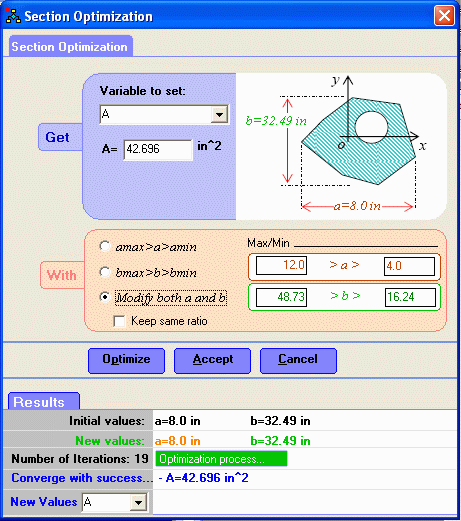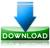# Complex Section Optimization

The Section Optimization component is used to optimize the geometry boundaries in order to get a desired value of specific variable. The variables that we can use in the optimization process are:

• A: Area of the cross section
• Ixc: Moment of inertia about x axis through centroid
• Iyc: Moment of inertia about y axis through centroid
• Ioc: Polar moment of inertia about origin of the centroidal axes
• Stx: Elastic Modulus about x axis at top
• Sbx: Elastic Modulus about x axis at bottom
• Sty: Elastic Modulus about x axis at top
• Sby: Elastic Modulus about y axis at bottom
• Zx: Plastic Modulus about y axis
• Zy: Plastic Modulus about y axis
• rx: Radius of gyration about x axis
• ry: Radius of gyration about y axisDownload